Calculator

# CalculatorIt is an analog of a standard calculator, with the ability to retrieve data from a drawing, adding a result to a drawing, and the ability to manually write a formula for calculating

### CALL OPTIONS

• ModPlus palette
• Command line: _mpCalc

### ICON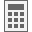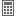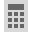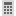### USING

In the upper left part of the window there is a button that opens the menu for selecting the work option - Calculator; Interpolation; Degree, slope: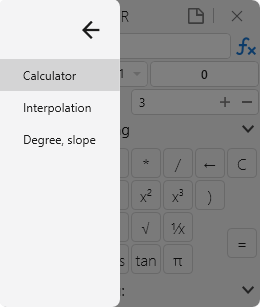Use transparaency - When setting is checked, the window will become transparent when the focus is lost

Calculator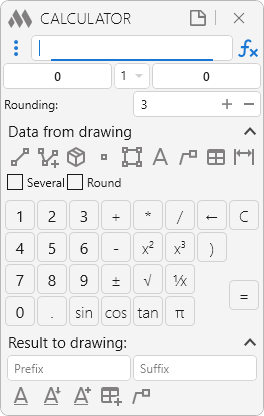Input field - field for entering the formula. After pressing the button "=" or on the keyboard of the button Enter the formula from the window will be calculated, and the result is displayed in the corresponding field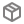- The context menu for inserting functions. For more information about the functions, see below in the table

Prefix - field for specifying the prefix. When adding a result to the drawing, the prefix will be added to the beginning of the result.

Output field - field for outputting the result of calculating the formula.

Suffix - field for specifying the suffix. When adding a result to the drawing, the suffix will be added to the end of the result.

Rounding - The indication of the number of decimal places to which the value in the output field will be rounded.

Группа Data from drawing contains functions for obtaining values from the drawing (the value is added to the end of the line of the input field). For some data retrieval options, it is possible to retrieve data from several objects by ticking the Several. Then when you select several objects from which you want to get the values, a window for selecting the sign will appear, which will be inserted between the values: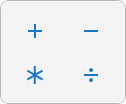Round - indicates whether the values obtained from the drawing are rounded to the specified number of decimal places.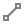- Measure the distance between two points Go to AutoCAD to query two points: First point: Specifying a point on the screen Second point: Specifying a point on the screen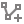- Get the sum of lengths from entities Switching to AutoCAD for selecting primitives: Select lines, polylines, arcs or circles: Selecting the appropriate entities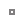- Get coordinates Go to AutoCAD to specify a point on the screen: Specify point: Specifying a point on the screen Select a coordinate [X/Y/Z]: Selecting the corresponding coordinate of the specified point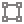- Get the area Go to AutoCAD to select an entity: Get the area [Polyline/Hatch]: The choice of the entity from which the area will be obtained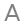- Get value from text Go to AutoCAD to select an entity: Select single-line or multi-line text: Select single-line or multi-line text Several mode: Select several single-line or multi-line texts: Select several single-line or multi-line texts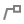- Get a value from the multileader Go to AutoCAD to select an entity: Select multileader: Select multileader Several mode: Select several multileaders: Select several multileaders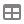- Get value from table cell Go to AutoCAD to select an entity: Select table: Select table Select cell: Selecting a cell in a table Several mode: Select table: Select table First corner of the frame: Selecting the cell in the table, which will be the first corner of the selection frame Second corner of the frame: Select a cell in the table, which will be the second corner of the selection frame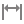- Get the value from the size Go to AutoCAD to select an entity: Select dimension: Select dimension Several mode: Select several dimensions: Select several dimensions

The group of control buttons includes buttons for adding/removing of digits in the input field, for the purification of the input field, for calculating the formula as well as certain functions. A complete list of buttons and functions (including those missing in the group of control buttons) is given in the table:

 Buttons 0...9 Adding to the end of the text in the input field a digit corresponding to the pressed button . Adding a dot at the end of the text + Adding a plus sign to the end of the text in the input field - Adding a minus sign to the end of the text * Adding the "multiply" sign to the end of the text in the input field / Adding the "divide" sign to the end of the text x² Raising the second power of a number from the input field or result field (if there is no value in the input field) x³ Raising the third power of a number from the input field or result field (if there is no value in the input field) √ Extracting a square root from a number from the input field or the result field (if there is no value in the input field) ± Adding/deleting the minus sign as a result 1/х Divide the unit by the number from the input field or the result field (if there is no value in the input field) sin Returns the sine of the specified number from the input field or the result field (if there is no value in the input field) tan Returns the tangent of a given number from the input field or the result field (if there is no value in the input field) π The display in the input field of the number "pi" ← Erasing one (last) character in the input field C Clear input field and output field = Calculating the formula in the input field and displaying the result in the output field Functions not found in the group of control buttons Abs(x) Returns the absolute value of the specified number Acos(x) Returns the angle whose cosine is equal to the specified number Asin(x) Returns the angle whose sine is equal to the specified number Atan(x) Returns the angle whose tangent is equal to the specified number Ceiling(x) Returns the smallest integer greater than or equal to a given number Exp(x) Returns e raised to the specified degree Floor(x) Returns the largest integer less than or equal to a specified number IEEERemainder(x,y) Returns the remainder of dividing the specified number by another specified number Log(x,y) Returns the logarithm of the specified number Log10(x) Returns the logarithm with base 10 of the specified number Max(x,y) Returns the larger of the two specified numbers Min(x,y) Returns the smaller of the two specified numbers Pow(x,y) Returns the specified number raised to the specified degree Round(x,y) Rounds a value to the nearest integer or specified number of decimal places Sign(x) Returns the value that specifies the sign of a number Truncate(x) Computes the integer part of a number

The group Result to drawing contains functions for inserting the result from the output field into the drawing: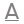- Insert result as a single-line text Go to AutoCAD to insert an entity: Insertion point: Specifying a single-line text insertion point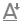- Add result to existing one-line text (with content replacement) Go to AutoCAD to select an entity: Select single-line text: Select one-line text to replace its contents- insert result into a table cell Go to AutoCAD to select an entity: Select table: Select table Select cell: Selecting a cell in a table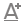- Add result to multiline text Go to AutoCAD to select an entity: Select multiline text: Selecting multi-line text to add results to it- insert result in the form of a multileader Go to AutoCAD to specify points: Specify point: Specifying the location of the leader arrow Insertion point: The location of the leader shelf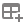- Opens a journal in which the last calculations are displayed:If you select in the calculation journal, it will be re-entered in the input field. The journal is kept only in the current session of the function. When you close and restart the function, the journal will be cleared- Clearing the calculations journal

Interpolation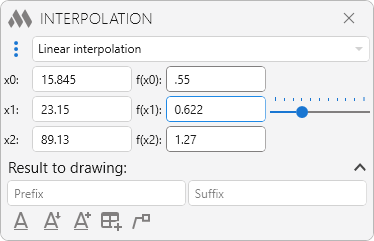Three options (selection in the drop-down list) - linear interpolation, linear extrapolation of the smaller and linear extrapolation of the larger. Calculation of the value occurs "on the fly" - i.e. when you enter the required values into cells

Degree, slope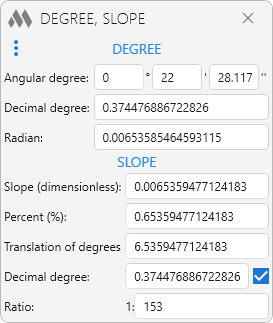Allows you to translate degrees and slopes into different values. Calculation of the values occurs "on the fly" - i.e. when you enter a value in any field